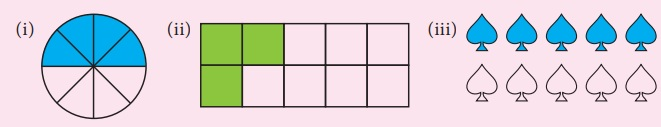Home | | Maths 7th Std | Number System

# Number System

Learning Objectives ● To recall the decimal notation and to understand the place value in decimals. ● To learn the concepts of decimals as fractions with denominators of tens and its powers. ● To represent decimal numbers on number line.

Chapter 1

NUMBER SYSTEMLearning Objectives

● To recall the decimal notation and to understand the place value in decimals.

● To learn the concepts of decimals as fractions with denominators of tens and its powers.

● To represent decimal numbers on number line.

Recap

Decimal Numbers

Kala and Kavin went to a stationery shop to buy pencils. Their conversation is given below.

Kala : What is the price of a pencil?

Shopkeeper : The price of a pencil is four rupees and fifty paise.

Kala : Ok sir. Give me a pencil.

Kavin : We usually express the bill amount in rupees and paise as decimals. So the price of a pencil can be expressed as ₹4.50. Here 4 is the integral part and 50 is the decimal part. The dot represents the decimal point.

(After a week of time in the class room)

Teacher : We have studied about fractions and decimal numbers in earlier classes. Let  us recall decimals now. Kala and kavin, can you measure the length of your pencils?

Kavin : Both the pencils seem to be of same length. Shall we measure and check?

Kala : Ok Kavin. The length of my pencil is 4 cm 3 mm (Fig. 1.1).Kavin : Length of my pencil is 4 cm 5 mm  (Fig. 1.2).

Kala : Can we express these lengths in terms of centimetres?

Kavin : Each centimetre is divided into ten equal parts known as millimetres. Do you remember that we have studied about tenths. I can say the length of my pencil as 4 and 5 tenths of a cm.

Kala : Since 1 mm = 1/10 cm or one tenth of a cm, it can be represented as 4.5 cm.

Kavin : Then the length of your pencil is 4.3 cm. Is it right?

Teacher : Both of you are right. Now we will further study about decimal numbers.

Try these

1. Observe the following and write the fraction of the shaded portion and mention in decimal form also.(i) 4/8 = 0.5

(ii) 3/10 = 0.3

(iii) 5/10 = 0.5

2. Represent the following fractions in decimal form by converting denominator into ten or powers of 10.3. Give any two life situations where we use decimal numbers.

The length of shirt cloth is 2.50 m.

The cost of one packet of chocolate is ₹ 20.60

Introduction

Consider the following situation. Ravi has planned to celebrate pongal festival in his native place Kanthapuram. He has purchased dress materials and groceries for the celebration. The details are furnished below.

Bill-1Bill-2What do you observe in the bills shown above? The prices are usually represented in decimals. But the quantities of length are represented in terms of metre and centimetre and that of weight are represented in terms of kilograms and grams. To express the quantities in terms of higher units, we use the concept of decimals.

MATHEMATICS ALIVE- Number system in Real LifeTags : Term 2 Chapter 1 | 7th Maths , 7th Maths : Term 2 Unit 1 : Number System
Study Material, Lecturing Notes, Assignment, Reference, Wiki description explanation, brief detail
7th Maths : Term 2 Unit 1 : Number System : Number System | Term 2 Chapter 1 | 7th Maths